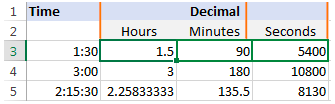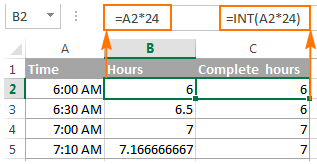Get instant live expert help with Excel or Google Sheets“My Excelchat expert helped me in less than 20 minutes, saving me what would have been 5 hours of work!”

#### Post your problem and you’ll get expert help in seconds.

Your message must be at least 40 characters
Our professional experts are available now. Your privacy is guaranteed.

# How to convert decimal time to Minutes and hours in Excel – Excelchat

When working with Excel, we might need to convert decimal time to minutes, hours or even seconds. This is just to make it easy to use the time in other calculations. At the same time, we might have to convert minutes to decimal, hours to decimal or seconds to decimal. In this post, we shall learn how to convert decimal time to minutes, hours and seconds.

Generally, we shall look at three different ways in which we can convert decimals into time. These include arithmetic operation, CONVERT function and combination of three different Time functions.

Usually, multiplying the original time value by the number of hours, minutes or seconds is the easiest way to convert decimal time value.

If you have time value in decimals and you want to convert it into hours, all you have to do is multiply it by 24. Note that 24 here represents 24 hours, the number of hours in a day.

In the event that you have time in decimals and you want to convert the decimal to minutes, then you can multiply it by 1440, which is the number of minutes in a day.

For those cases where we are supposed to convert the decimal time to seconds, we can simply multiply the decimal time by the number of seconds in a day, which is 86400.Figure 1: How to convert decimal time to hours, minutes and seconds

### How to convert decimal time to hours using arithmetic calculations

As stated above, changing time from decimal to hours we simply need to multiply the decimal time by the number of hours per day;

`=A1*24`

Now if you want to get the number of hours through arithmetic calculations simply embed the above formula into an INT function. This function will simply help you remove the decimal part of the time.Figure 2: Converting decimal time to minutes using INT function

### Converting decimal time to hours using the CONVERT function

We can also use a convert function to get the number of hours from decimal time.

`=HOUR (A1) + MINUTE (A1)/60 + SECOND (A1)/3600`

If we want to convert hours to a decimal chart, we can do a reverse of what we have done in the process above. Where we have multiplied, we shall divide, and where we have divided, we shall multiple.

Converting time to a decimal chart is very essential if you want to use it for other calculations. Also, if you know that the decimal time you have will be used by someone else, who might not know what it actually means then converting the time to hours and minutes will simplify the work for him/her.

## Conclusion

The process of decimals to minutes conversion ought not be as difficult as many would want to imagine. As long as you have the basic tips on how to convert decimal to hours, minutes or second, then you are able to come up with a decimal to time chart that can serve your purposes right.

## Instant Connection to an Expert through our Excelchat Service

Most of the time, the problem you will need to solve will be more complex than a simple application of a formula or function. If you want to save hours of research and frustration, try our live Excelchat service! Our Excel Experts are available 24/7 to answer any Excel question you may have. We guarantee a connection within 30 seconds and a customized solution within 20 minutes.